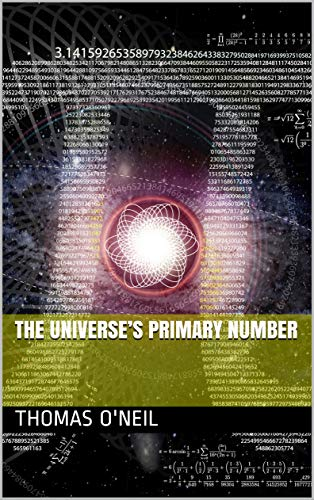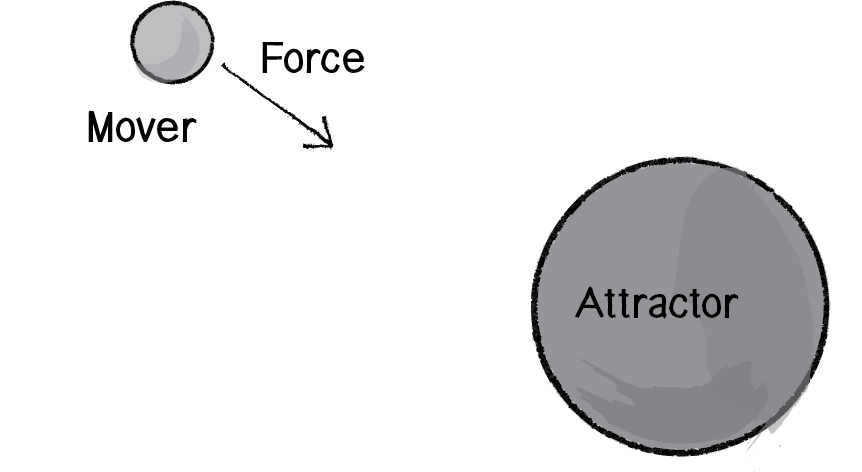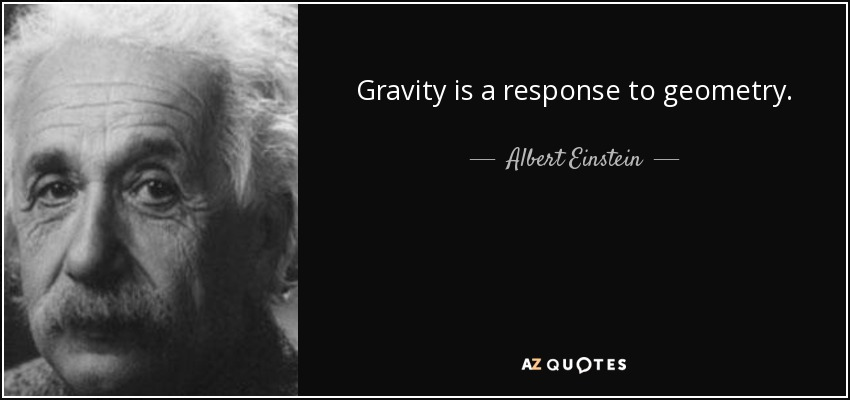Join the Unexplained Mysteries community today! It's free and setting up an account only takes a moment.# The Universe's Primary Number

## Recommended PostsPosted (edited)

To all a miracle

H2O divided to form land} 1.9e+63 TNT / 3.14 = mc^2

Energy minus Hydrogen} 6.050955414012739e+62 TNT = mc^2

Energy of Hydrogen} 1.2101910828025478e+63 TNT = mc^2

Total Amount of Energy in the Universe }1.9e+63 TNT = mc^2

Approximately 73% of the mass of the visible universe is in the form of hydrogen. Helium makes up about 25% of the mass, and everything else represents only 2%."

As I have just shown through Pi the mass of Hydrogen, I can now show the average daylight hours. I will do this through Pi and the speed of light along with a time constant I found through the transcendental number e.  The first unit of time can be found in my transcendental e python program and is 33554432.  So what you need to do to do this I will list the numbers.

•    Speed of Light = 299 792 458 m / s you need this number

•    Time constant from e = 33554432 you need this number

To calculate Time e exponential growth = b^((n)+(r))

b = base n = number r = growth rate so 2^ ((1) + (24)) =33554432

Calculation for e = 2^ ((1) + (360/15)) = 33554432

•    299 792 458 x .314 = 94,134,831.812 you need this number

•    94,134,831.812/3.14 = 29,979,245.8

•    29,979,245.8/3.14 = 9,547,530.50 you need this number

299,792,458

94,134,831.812

9,547,530.50

+______________________

403,474,820.312

403,474,820.312 / 33554432 = 12.02 daylight hours

Therefore Pi 3.14 was involved in the process of dividing the light and this would show that our dimension was cut from 3.14!

Transcendental e Time python Code:

```	import time
print('''This number 33554432.0 after typing 1 in represents 1 days of
creation.
________________________________________ ''')
while True:

n = int(input("Enter a time in hours: "))
start_time = time.time()

p = (pow(2,n))

t = (pow(2,((n +(((360)/(((15)))))))))

print(p,'one unit')
print(t,'Trancendental time')
e = int(time.time() - start_time)
print('{:02d}:{:02d}:{:02d}'.format(e // 3600, (e % 3600 // 60), e % 60))

```

If this miracle of logic shocks you I have published a book at Amazon under the title "The Universe's Primary Number".Gravity Waves are associated with Pi through a calculation below from a time division of 12 hours divided by 10,000

Pi is amazing at every level in its involvement with the universe and I believe in the below formula I have proved that Einstein is absolutely correct.  This proof through math with Pi of the geometric equivalent of what Einstein described as gravity represented as a space-time curvature may explain why its attractive.  Think of all the planets and stars as not being perfect spheres, because something at the atomic level with the fine structure constant is interacting with Pi thus enabling attraction. Space time is Warped and the equation does involve time below as-well as the fine structure constant.  Therefore the warp in space geometry is created by 314 and induces attraction.  314 is at the tail of the end result of the calculation below.

So a geodesic is the shortest path.  To show the mystery of attraction of gravity multiply these two.

Geodesic x 6.28000000000000314

Quote

“The mystery of gravity is that it is an attractive force –it's actually very weak compared to the other three main forces, but while the others can be both positive and negative and cancel each other out, gravity is attractive, and therefore cumulative, with no way to cancel it out.

I now move on to gravity and yes Pi seems to be involved with the mystery of gravities attraction using the Gravitational Constant.  Here is the formula:

To calculate 2π using 12/10,000 hours, the Gravitational Constant and the Fine Structure Constant

I will use this formula b^((n)+(r))

b = base n = number r = growth rate

The smallest, most precise measurement ever made required one of the largest scientific instruments ever constructed. Five years ago the Laser Interferometer Gravitational-Wave Observatory (LIGO) detected

a ripple in spacetime that was just one ten-thousandth the width of a proton—a technical tour de force tantamount to pinpointing the distance to the nearest star to three one-thousandths of a centimeter. The Lilliputian ripple was a gravitational wave, a distortion in the fabric of the cosmos generated by the collision of two black holes more than a billion light-years from Earth.

2^ ((.00000000006674*.0072973*(12 hours/10000)) + (1)) = 2.000000000000001

I know this is not a 360 but here we go 2.000000000000001x3.14=6.28000000000000314

The tail is 314 and so Gravities Attraction relates to Pi π! And I speculate gravities mystery attraction engine is geometric!

Einstein, in the context of his theory of General Relativity (GR), has interpreted gravity as a geometric property, space-time curvature. It has been shown that, using Einstein's point of view, one can interpret more physical phenomenae than using Newton's one.

Python code will show as weight of one body increases force goes up:

```	# Python Program to Calculate Gravitational Force

# setting value of Gravitational Constant
G = (.00000000006674)

while True:

m1 = int(input('Enter weight of first body (Kg): '))
m2 = int(input('Enter weight of second body (Kg): '))
r = int(input('Enter distance (m): '))

# Calculating Gravitational Force
F = (((G * m1) * m2) / r**2)

# Displaying result
print(m1,'Weight of First Body Kg')
print(m2,'Weight of Second Body Kg')
print('{0:.100f}'.format(F),'Gravitational Force')
print('{0:.14f}'.format(G),'Gravitational Constant')
```

[USER=78319]@ALL[/USER] The significance of what I did with Pi and Gravity

Read this carefully 10,000th is important

Five years ago the Laser Interferometer Gravitational-Wave Observatory (LIGO) detected a ripple in spacetime that was just

one ten-thousandth the width of a proton—a technical tour de force tantamount to pinpointing the distance to the nearest star to three one-thousandths of a centimeter.

I obtained this number 6.28000000000000314 from the below calculations

To calculate 2π using 12/10,000 hours, the Gravitational Constant and the Fine Structure Constant I will use this formula b^((n)+(r))  b = base n = number r = growth rate

2^ ((.00000000006674*.0072973*(12 hours/10000)) + (1)) = 2.000000000000001

I know this is not a 360 but here we go 2.000000000000001x3.14=6.28000000000000314

So here me out, I set out knowing the information from the quote above from Scientific American that the Gravity Wave measured 1/10000 of the width of a proton.  This is an actual number representation of the Ligo Gravity Wave that was measured from a black hole merger 1.4 billion light years away.

Therefore if my Pi formula is accurate for 6.28000000000000314 then the Ligo Gravity Wave should divide evenly with no decimal and it should be straight forward with no fraction involved in the output result.

So here are the numbers:

proton diameter = .000000000000010

My number = 6.28000000000000314

Ligo Gravity Wave Measurement = 0.00000000000000000100000000000000007154242405462192450852805618492324772617063644020163337700068950653076171875

I was shocked no fraction:

6.28000000000000314 /

0.00000000000000000100000000000000007154242405462192450852805618492324772617063644020163337700068950653076171875 =

2000000000000313856.0 ONeil_Pi_Gwave_measured! No fraction Pi is correct for the Mystery of Gravity

```	# Reading weight and distance
while True:
G = (.00000000006674)
proton_width = (.00000000000001)

gravity_wave = (proton_width/10000)

ONeil_Pi_Gwave_measured = (2.000000000000314/(proton_width/10000))
m1 = int(input('Enter weight of first body (Kg): '))
m2 = int(input('Enter weight of second body (Kg): '))
r = int(input('Enter distance (m): '))

# Calculating Gravitational Force
F = (((G * m1) * m2) / r**2)

# Displaying result
print(m1,'Weight of First Body Kg')
print(m2,'Weight of Second Body Kg')
print('{0:.100f}'.format(F),'Gravitational Force')
print('{0:.14f}'.format(G),'Gravitational Constant')
print('{0:.110f}'.format(gravity_wave),'Gravity Wave')
print('{0:.99f}'.format(proton_width),'Proton Width')
print('{0:.1f}'.format(ONeil_Pi_Gwave_measured),'ONeil_Pi_Gwave_measured')

```

Edited by Tom O'Neil
•2

##### Share on other sites42

##### Share on other sites1 hour ago, Tom O'Neil said:

H2O divided to form land} 1.9e+63 TNT / 3.14 = mc^2

Meaningless number.  How are you working out the energy of land?

##### Share on other sitesTo All

"

"

I don't use Luminosity at all to measure the distance to the Black Hole Merger from the Ligo Gravity Wave

With the calculations below I have found the exact distance for the “LIGO’s Gravity wave source of the Black hole merger on September 14, 2015” through gravities geometry.  Ligo’s estimated the source distance of the Black Hole Merger through a method called luminosity which is known to be an approximation of 1,271,400,000 light years.  My method to find the source distance to the black merger utilizes a gravitational geometric number (6.28000000000000314) to pinpoint it at 1,262,599,571.4 light years.  Ligo over shot the distance to the Black Hole merger by 8,800,428.5 light years which shows luminosity is not exact.  You can see in my python code the calculations are here:

Here Einstein showed that the Luminosity of Black Holes is an approximation of distance in its Linear use:

Very shortly after announcing the general theory of relativity in , Albert Einstein realized that a linearized version of his equations resembles the wave equation (Einstein 1916). The solution is interpreted as a short-wavelength, time-varying curvature deformation propagating with the speed of light on an otherwise slowly-varying, large-scale curvature background (a gravitational-wave \"ripple\" propagating through the four-dimensional spacetime); from the point of view of a metric tensor, it represents a small perturbation of a stationary background metric. Linear approximation corresponds to the waves propagating in the far-field limit. By exploiting the gauge freedom of the theory one may show that the solution has features similar to electromagnetic waves: it is a transverse wave which may be polarized (has two independent polarizations). Over the next 40 years, during which Einstein changed his mind to argue against their genuineness, a controversy persisted over the true nature of gravitational waves. Only in the late 50s and early 60s the works of Felix Pirani (1956), Herman Bondi (1957), Ivor Robertson and Andrzej Trautman (1960) convincingly showed that gravitational waves are indeed physical phenomena that carry and deposit energy.

with the use of Kepler's third law (GM = a3 ω2) in the second equation. The expression in brackets represents the strain tensor hij in the non-relativistic quadrupole approximation (Einstein 1918). Similarly, the luminosity L (the rate of energy loss in gravitational waves, integrated over a sphere at a distance r) should be proportional to h2 r2 and some power of ω. From dimensional analysis one has

In addition to precisely measuring the Hubble constant, cosmological observations would help determine the distances to galaxies, thus contributing to building the standard 'distance ladder' (calibrating electromagnetic standard candles), establish the distribution of galaxies and voids, characterize the evolution of the dark energy and mass density of the Universe, mass distribution through the gravitational lensing, as well as the chemical evolution effects i.e., establishing the onset of star formation (Królak and Schutz 1987, Sathyaprakash and Schutz 2009). The truly multi-messenger era of astronomy is just beginning.

Black Hole Merger Source Distance Approximation from Luminosity

(1000000*3.26(410(180-160)))
=
1,271,400,000 light years

Black Hole Merger Source Distance from Gravity’s Geometry
((((2.000000000000001/(.00000000000001/10000))*86400*365)/(6.28000000000000314-(2.000000000000001/2)))/ 9461000000000000)
=
1,262,599,571.4 light years

On September 14, 2015, LIGO's interferometers in Livingston, LA and Hanford, WA made the world's first direct detection of gravitational waves, heralding a new era in astronomical exploration. The gravitational waves detected by LIGO on that day were generated by two black holes colliding and merging into one nearly 1.3 BILLION light years away!

https://www.ligo.caltech.edu/page/facts

“This pdf gives the Black Hole Merger Luminosity distance of the Ligo Gravity Wave Source
https://physics.aps.org/featured-article-pdf/10.1103/PhysRevLett.116.061102

Here is the python code for the Black Hole merger distance from the Ligo Gravity Wave:

```import time
print('''Two to the power of Exponential growth of Gravities
Constant <G> multiplied by the fine structure contant multiplied
by 12 hours divided by ten thousand plus one.
________________________________________ ''')
start_time = time.time()
G_constant = (.00000000006674)
proton_width = (.00000000000001)
gravity_wave = (proton_width/10000)
G = ((pow(2,((1 +(((.00000000006674*.0072973*.0012)*((( 1 ))))))))))
Gravities_Geometry = (G*3.14)
Distance_to_Gravity_Waves_Source = ((((2.000000000000001/(proton_width/10000))*86400*365)/(6.28000000000000314-(2.000000000000001/2)))/9461000000000000)
Ligos_approximation_Black_hole_merger_from_luminosity = (1000000*3.26*(410-(180-160)))
difference = (Ligos_approximation_Black_hole_merger_from_luminosity-Distance_to_Gravity_Waves_Source)
print('{0:.14f}'.format(G_constant),'Gravitational Constant')
print('{0:.99f}'.format(proton_width),'Proton Width')
print('{0:.110f}'.format(gravity_wave),'Gravity Wave')
print(G,'G as exponential growth')
print('{0:.15f}'.format(Gravities_Geometry),'Gravities_Geometry')
print(Ligos_approximation_Black_hole_merger_from_luminosity,'Ligos_approximation_Black_hole_merger_from_luminosity in light years')
print('{0:.1f}'.format(Distance_to_Gravity_Waves_Source),'Distance_to_Gravity_Waves_Source in light years to black hole merger')
print(difference,'difference from Ligo luminosity and Gravity wave source in light years')
e = int(time.time() - start_time)
print('{:02d}:{:02d}:{:02d}'.format(e // 3600, (e % 3600 // 60), e % 60))
```

I think I follow that Einstein shows that Luminosity is a very close approximation for a distance measurement.  The youtube will show that Geometry is why objects should be measured with my revolutionary equation. I believe this is just what Ligo looking for and read this concluding sentence.

##### Share on other sitesBTW have you won that noble peace prize yet?

•1

##### Share on other sites## The Nikola Tesla 3 6 9 theory or the key to the universe. Hey Tom you may be interested in this theory, according to tesla by incorporating and understanding this sequence of numbers the Universe is an open book.

##### Share on other sitesI believe since luminosity is not an exactly accurate measuring stick this is just why the excitement is about gravity waves.  After thinking about what Einstein wrote regarding luminosity as in light is not accurate to use exactly for distances, because of the linear constraint I thought how I could explain this to make it simple.

Here I go:

1 ) Gravity Waves can extend out and their path is not effected by other masses, so the distance measurement is direct.

2) Where as Light when it is emitted its path bends around stars so when using luminosity as a measuring tool for huge distances the lengths will stretch in the measurement with angles associated due to the curvature of the light wave created by other masses such as stars, black holes and planets.  This extends the light distance measurement.  Therefore, like the Ligo Luminosity Black Hole Merger Source Distance vs. My Geometric Gravity Wave Black Hole Merger Source Distance one would see a longer distance in Ligo's measurement, because it depended on purely light to approximate the distance to the Black Hole merger.

This is why I feel Ligo really needs to look at my math & Astrophysicists as-well, because I believe I figured out an accurate way to measure distances using the gravity wave without luminosity. This method finally reveals not only that Einstein is 100% correct about Geometry for the mystery of Gravity, but it uncovers the Geometric Engine Constant for Gravities true Mystery Number that Warps Space Time.  The Gravity Geometric is(6.28000000000000314).  The video below does an excellent job at showing Gravity and its Geometric on display.

##### Share on other sites"H2O divided to form land".

##### Share on other sitesJust a question, Tom: do you also post as "Stellar", or did you just do a copy and paste?